# Sizing the Inductor of Buck Converter and Setting its Operation

One of the most important parts of a buck converter is the inductor. Sizing the inductor of buck converter and setting its operation mode makes the converter function correctly. The shape and the magnitude of current of the inductor are dictated by the inductance of the inductor itself. Therefore choosing the right inductor value is very important. Sizing the inductor of buck converter and setting its operating mode is actually not a difficult task to do. You only need to understand how a buck converter works and how the waveforms look like. However, these may be too much to anybody who is not mastering or do not have any background on power electronics. This article is a good guide for you to successfully complete the job by your own and obtain a correctly functioning buck converter.

The inductor current of a buck converter is can be classified in three types; continuous, discontinuous or boundary. A continuous current means that the minimum level of the inductor current waveform is never touching zero in any switching period. A discontinuous current is the other way around. The minimum level of the inductor current is touching zero before the next PWM high or Ton occurs. In boundary current, the inductor current waveform minimum level is always at zero every switching cycle.

## Sizing the Inductor of Buck Converter to Operate in Continuous Conduction Mode (CCM)

``````

To ensure the operation of the converter in the CCM region, the inductor must be big enough such that its energy will not be depleted until the next charging cycle occurs. When the inductor current is continuous, it will not go to zero at any switching cycle. Because of this, the inductor ripple current is small. A smaller ripple current corresponds to lower losses on the inductor as well as on the active power devices. Thus, this is more preferred for higher efficiency requirement. The drawback on setting the inductor current of buck converter to CCM is that it needs a larger inductance. A larger inductance means a bulky physical size and there is maybe a price difference.

A mathematical expression is provided below in sizing the inductor of buck converter to surely operate in the CCM region.

``

Set the ripple current di to not higher than 20% of the output load current to ensure a CCM operation. So, we can change the above equation with the one below

``````

( Duty – duty cycle of the converter derived at either boundary or CCM, Vin – input voltage, Vout – output voltage, Fsw- switching frequency, Iload – maximum load current)

## Sizing the Inductor of Buck Converter to Operate in the Boundary Mode

```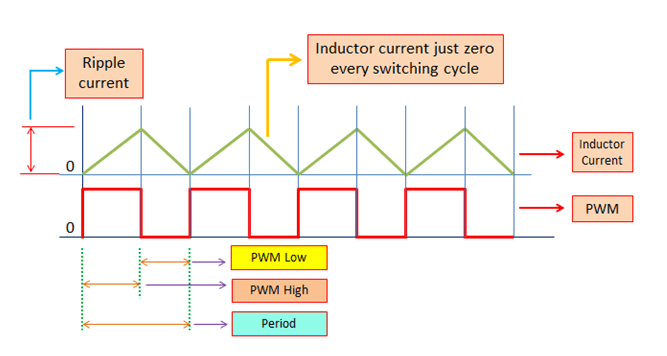```

Boundary mode is in between the CCM and DCM regions. So, sizing the inductor of buck converter less than that of the CCM value but higher than that of the DCM value will do. At the boundary mode, the inductor current is always at the zero level (just zero) every end of the switching period or during the next charging cycle. This operation is somewhat requiring a more complicated control algorithm since the controller needs to detect always the zero point of the inductor current waveform. There are some ICs that offer this type of algorithm nowadays. In terms of power losses, it has still higher losses than CCM but lower than DCM.

As mentioned, a boundary mode operation all throughout the entire load range requires a more complicated control algorithm (anyway nowadays there are several available solutions for this). Without dealing with any control mechanism here, just with the waveform of the inductor current, we can draw an equation that can determine when the buck converter’s operation go to the boundary, as below

`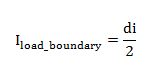`

di is given by the equation

`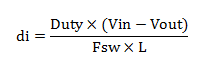`

Substitute di to the above equation

`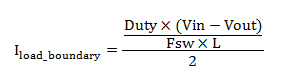`

So, the size of the inductor to make the buck converter operates at the boundary with a specific load current that is represented by the variable I

load_boundary is given by the equation below

`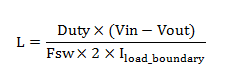`

(L – Inductance value, Duty – duty cycle, Vin – input voltage, Vout – output voltage, Fsw – switching frequency, Iload_boundary – the specific load where the converter is set to operate at the boundary)

Read Buck Converter Duty Cycle Derivation for very detailed duty cycle explanations.

## Sizing the Inductor of Buck Converter to Operate in Discontinuous Conduction Mode (DCM)

`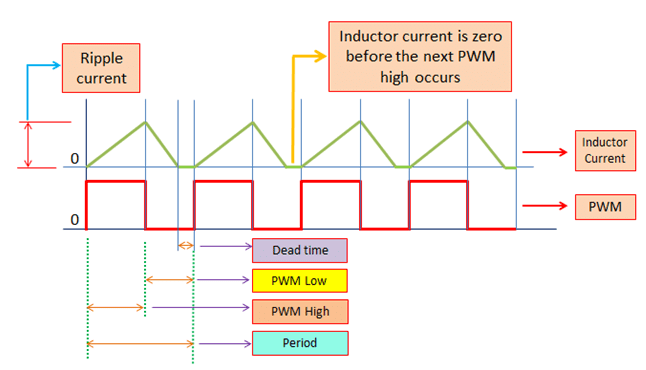`

A smaller inductor value will bring the buck converter operation to DCM. Buck converters especially with high power rating are not deliberately set to operate at DCM. Letting the current on the inductor to become discontinuous yields a very high peak value and the RMS currents of the inductor as well as the active devices are high. These correspond to high power losses that jeopardize efficiency and needs more rugged and thus expensive devices. The inductor ripple current is very high as well.

As illustrated in the above waveform, there is a dead time on the inductor current. This means that the energy on the inductor is already consumed before the next charging period occur or the next PWM high.

Although in normal cases buck converter is not set to operate in DCM, however, it will still enter a discontinuous current state at light load. For example, the full load current of a buck converter is 10. At this load, the buck converter is designed to have continuous inductor current and should not be the other way around. However, when the load reaches to 3A for instance, there is a tendency that the current will become discontinuous. Thus, sizing the inductor of buck converter is really very important and noted with high priority so that the load current when the converter starts to enter DCM is known and appropriate actions are performed ahead of any issues. Failing to do so, the efficiency may be affected because once the buck converter enters DCM; its RMS losses will increase. Stresses may also be a problem if not accounted.

Below is the equation use in sizing the inductor of buck converter to operate in DCM region. The more the value of L becomes smaller than the right side expression will make the converter operation more discontinuous.

``````

## Some Useful Equations for Design Checking

### Inductor Ripple Current

The inductor ripple current is can be expressed using the equation below. You can use this for checking when you have the value of inductance known. This equation is derived when the inductor current is not entering the DCM region. So, this is not accurate to be used when the operation is DCM.

``````

In the above equation, Duty is the duty cycle. You must take note that this duty cycle is derived when the inductor current is at the boundary or at CCM mode. Vin, Vout, Fsw and L are input voltage, output voltage, switching frequency and inductance respectively.

### Ideal Duty Cycle

The ideal duty cycle of a buck converter with a CCM and boundary mode currents is can be expressed as

``````

The above equation is can be re-written as

``````

Inductor RMS Current

The RMS current for CCM and boundary modes is

```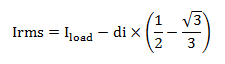```

### Inductor Peak Current

The maximum inductor current is can be computed using the equation below:

``````

### Accounting the DCM Region

What we tackled so far are valid for both CCM and boundary currents only. These two current modes are easier to derive their equations. The discontinuity or the presence of a dead time in the DCM current makes it relatively difficult to deal with. Design engineers are actually not designing the buck converter to operate at DCM current at normal operation (except may be for a special requirement). Thus, obtaining the exact DCM current level and formulating an equation for it is not anymore necessary. It is enough to know when the buck converter starts to enter DCM region. The load current when the inductor current of buck converter become discontinuous is given by the equation

``````

Where di in the above equation is the same to the di derived previously. During the design stage, you as a designer need to set a minimum load current when the converter starts to operate in the discontinuous region. Making the operation continuous across all loading conditions means a very large inductor. For instance, the full load current of a buck converter is 20A, the usual designs make the converter operates at CCM current down to 5A and below this it’s going to operate in the DCM region. This is alright as long as designed to be like this and thus all the parameters that might be affected are already been considered. Making the operation CCM down to 0.1A requires a very large value inductor.

## Example Design

Vin = 12V, Vout =3.3V, Fsw = 200 kHz, Iload_max = 25A, L = 10µH

Compute for:

a. Ideal duty cycle, b. ripple current, c. Peak current , d. RMS current, e. Boundary current

### a. Ideal duty cycle

```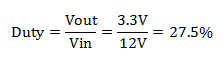```

### b. Ripple current

```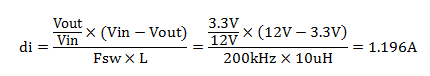```

### c. Peak Current

``````

### d. RMS Current

``````

### e. Boundary Current

```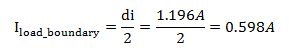```

The computed ripple current is very small and the converter will only enter DCM current at 0.6A. This is relatively a very small load current already. A 10uH inductor that can sustain 25A current (actual current rating should be above 25A) is bulky. If board space is a concern, the 10uH inductor is simply too big. A ripple current of 20% to the maximum load current is still a good level (I also adopted this on my designs). So, an inductor with an inductance of 2.2uH is still okay to use.
The new ripple current will be

``````
```The new peak current will be```

The new RMS level will be

```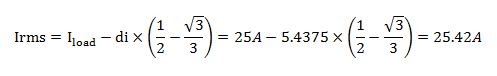```

The new boundary current will be

``````

Available 10uH, 37A inductors online

``````

Available 2.2uH, 40A inductor online

`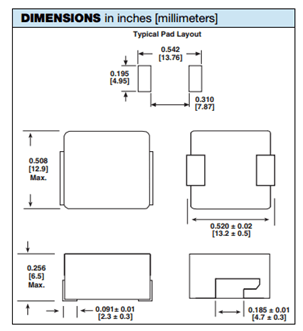`

There is a very huge size disparity between the 10uH and 2.2uH inductor that can handle the same maximum current.

## Summary

Sizing the inductor of buck converter is critical to the operation of the converter. A bigger inductor will surely make the operation CCM. In CCM the ripple current is low. The RMS currents are low as well thus the power losses are small. The drawback is the physical size of the inductor. In usual application, buck converter is set at CCM at higher loads and let it operate in the DCM at lower load. This is a more practical approach to optimize the design in terms of the operation mode and the physical size of the inductor. Just ensure that when the buck converter enter DCM region, there will be no associated issues.

## One comment

This site uses Akismet to reduce spam. Learn how your comment data is processed.